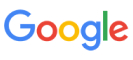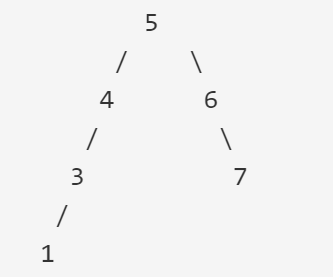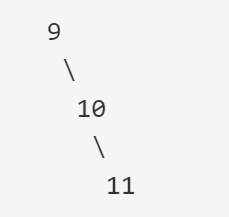New update is available. Click here to update.
Topics

# Guess Price

Easy0/40
Average time to solve is 10m+1 more companies

## Problem statement

There is no salesperson in the store. So you are supposed to guess the price of a given BST, which is the minimum value in its nodes.

Detailed explanation ( Input/output format, Notes, Images )
Constraints -
``````1 <= 'T' <= 10
1 <= 'N' <= 10^5
1 <= A[i] <= 10^6 or A[i] = -1,  i ∈ (1, N)
Note - The sum of 'N' over all test cases does not exceed 2 * 10^5.

Time Limit: 1 sec
``````
Sample Input 1:
``````2
13
5 4 6 3 -1 -1 7 1 -1 -1 -1 -1 -1
7
9 -1 10 -1 11 -1 -1
``````
Sample Output 1
``````1
9
``````
Explanation for Sample Input 1:
``````For Case 1:
````````````Given input looks like the tree drawn above. It can be seen that the minimum value in the tree is 1.

For Case 2:
````````````    Given input looks like the tree drawn above. It can be seen that the minimum value in the tree is 9.
``````
Sample Input 2:
``````2
7
20 8 22 -1 -1 -1 -1
15
40 9 50 4 12 -1 -1 -1 -1 10 14 -1 -1 -1 -1
``````
Sample Output 2:
``````8
4
``````Console